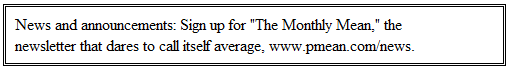P.Mean: Ordinal entropy (created 2010-03-11).I have been using the concept of entropy to evaluate a sperm morphology classification system and to identify aberrant records in large fixed format text files. Some of the data I have been using in these areas is ordinal with three levels, normal, borderline, and abnormal. In all of my work so far, I have treated all three categories symmetrically. So, for example, the entropy of a system where 50% of the probability is associated with normal and 50% is associated with borderline is 1. The entropy of a system where 50% of the probability is associated with normal and 50% is associated with abnormal is also 1. It has always bothered me a bit because it seems that the second case, where the probabilities are placed at the two extremes, should have a higher level of entropy. Here is a brief outline of how I think entropy ought to be redefined to take into account the ordinal nature of a variable.

Here are some definitions. If you have a probability, p, then the surprisal is defined asIf p=1/32, then the surprisal is 5, which corresponds to an event that is as surprising as getting five consecutive heads on the flip of a coin. If an event has zero probability, the surprisal is infinity. We need to tread with a bit of care around this fact.

If an event has probability 1, then the surprisal is zero. This is a quantification of the fact that an event that always occurs offers no surprise.

Now assume that n raters are asked to provide a categorical rating to an object. The values of the categorical rating are C1, C2, ..., Ck. Let n1, n2, ..., nk be the number of raters who selected each of the particular categories and defineThen the entropy is defined asWhat if the categories C1, C2, ..., Ck are ordinal? Then an alternative approach is to compute the entropies for the cumulative probabilities.The ordinal entropy is the sum of these individual entropies. An equivalent definition would use the average rather than the sum, and I have not decided which makes more sense yet.

Let's look at some simple examples. Suppose a distribution has 50% probability for C1, 50% for C2 and 0% for C3. The ordinal entropy would be the entropy calculated from the first cumulative probability and its complements (p1=0.5 and p2+p3=0.5) and from the second cumulative probability and its complement (p1+p2=1.0 and p3=0.0). The entropies are 1.0 and 0.0 respectively so the ordinal entropy is 1.0.

Suppose a distribution has 50% probability for C1, 0% for C2 and 50% for C3. The first cumulative probability and its complement are p1=0.5, p2+p3=0.5. The second cumulative probability and its complement are p1+p2=0.5 and p3=0.5. The entropies are both 1.0, so the ordinal entropy is 2.0.

Notice that splitting the two probabilities between the two extreme categories produces twice as much entropy as splitting between one extreme and a middle category.

The surprisal value for ordinal entropy is simply the sum of surprisals associated with the respective cumulative probabilities. The ordinal surprisal for a probability p3 associated with the third of five ordinal categories would simply be the sum of the surprisals associated with p2+p3+p4+p5, p3+p4+p5, p1+p2+p3, p1+p2+p3+p4.

Here's a display of all the cumulative probabilities and their complements. The surprisal for each row is the choice of whichever between the cumulative probability and the complement includes p3.Here are some graphs that contrast the behavior of entropy and normal entropy for a variable with three levels. Here is a contour plot for entropy.In this graph, the lower left corner of the triangle corresponds to p1=100%, the corner in the top middle corresponds to p2=100%, and the corner in the lower right corresponds to p3=100%. Red represents regions where the entropy is less than 0.3, orange where entropy is between 0.3 and 0.6, etc. The white region in the center of the triangle corresponds to entropy between 1.5 and 1.8. The smallest values for entropy, of course, are near the corners where the probabilities are concentrated almost exclusively with one category. The maximum entropy corresponds to an equal spreading of probability among the three categories (p1=1/3, p2=1/3, p3=1/3). This point appears at the center of the graph and the value for the maximum entropy is 1.58.

Here is the contour plot for ordinal entropy.The ordinal entropy is not too different from entropy at the corners. There is an additional region in black, which corresponds to ordinal entropy values between 1.8 and 2.1. The maximum ordinal entropy (2.0) does not occur in the middle of the triangle at p1=1/3, p2=1/3, p3=1/3, but rather at the middle of the bottom of the triangle, corresponding to p1=1/2, p2=0, p3=1/2.

The concept of ordinal entropy is not too technical, so I suspect someone has done this before. When I have time, I'll add some discussions about ordinal entropy and see if I can relate it to previous publications.This work is licensed under a Creative Commons Attribution 3.0 United States License. This page was written by Steve Simon and was last modified on 2017-06-15. Need more information? I have a page with general help resources. You can also browse for pages similar to this one at Category: Information theory.